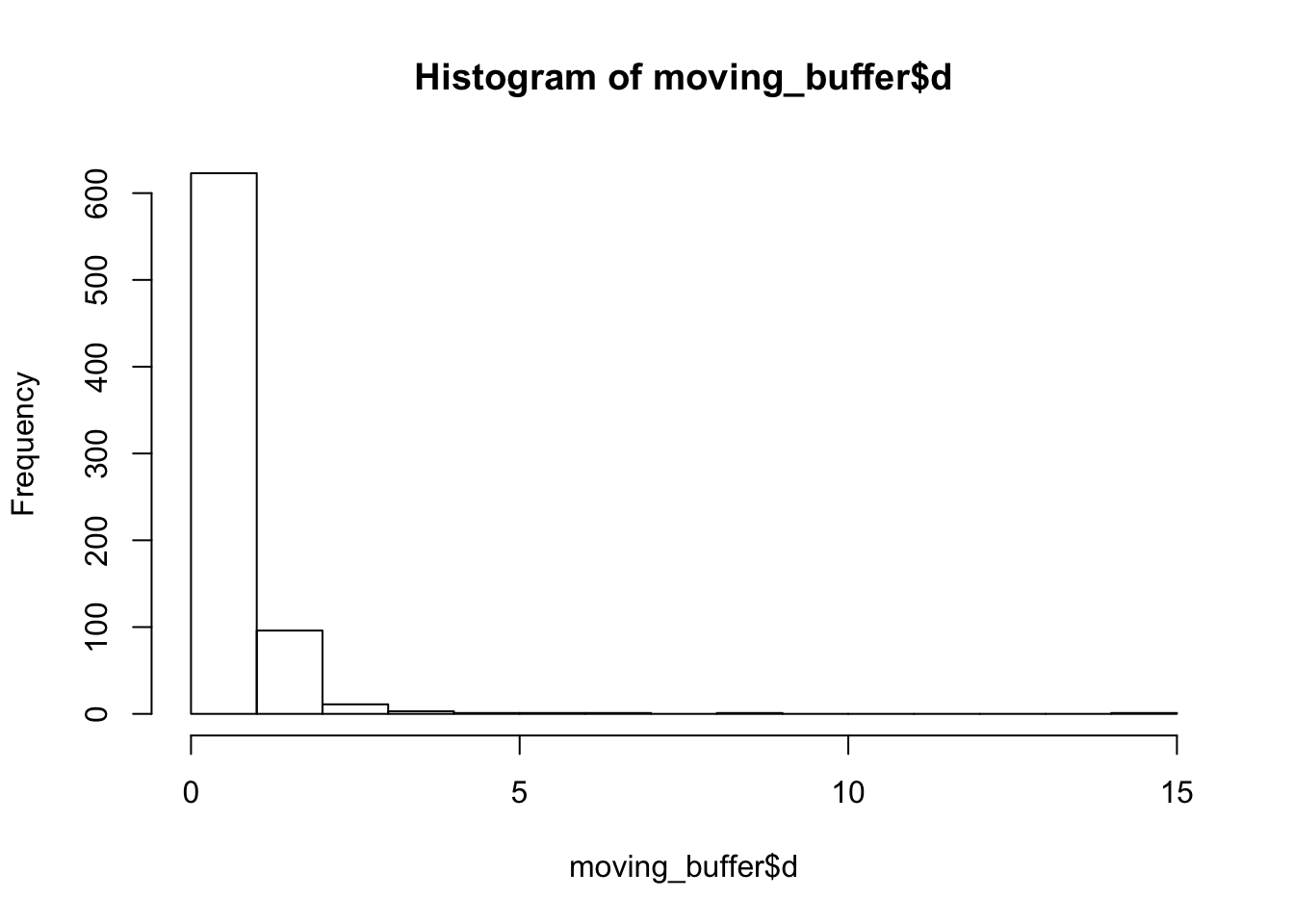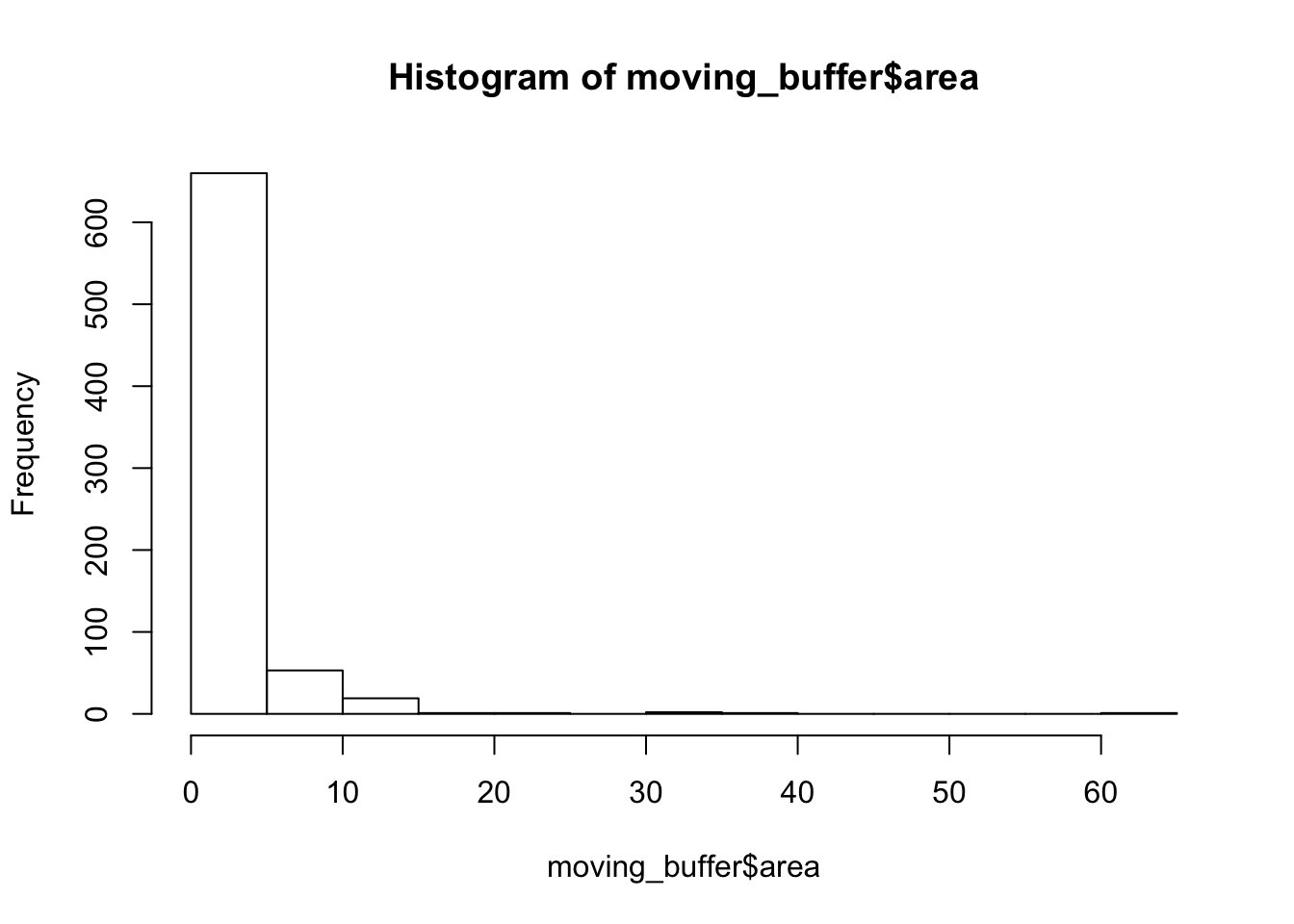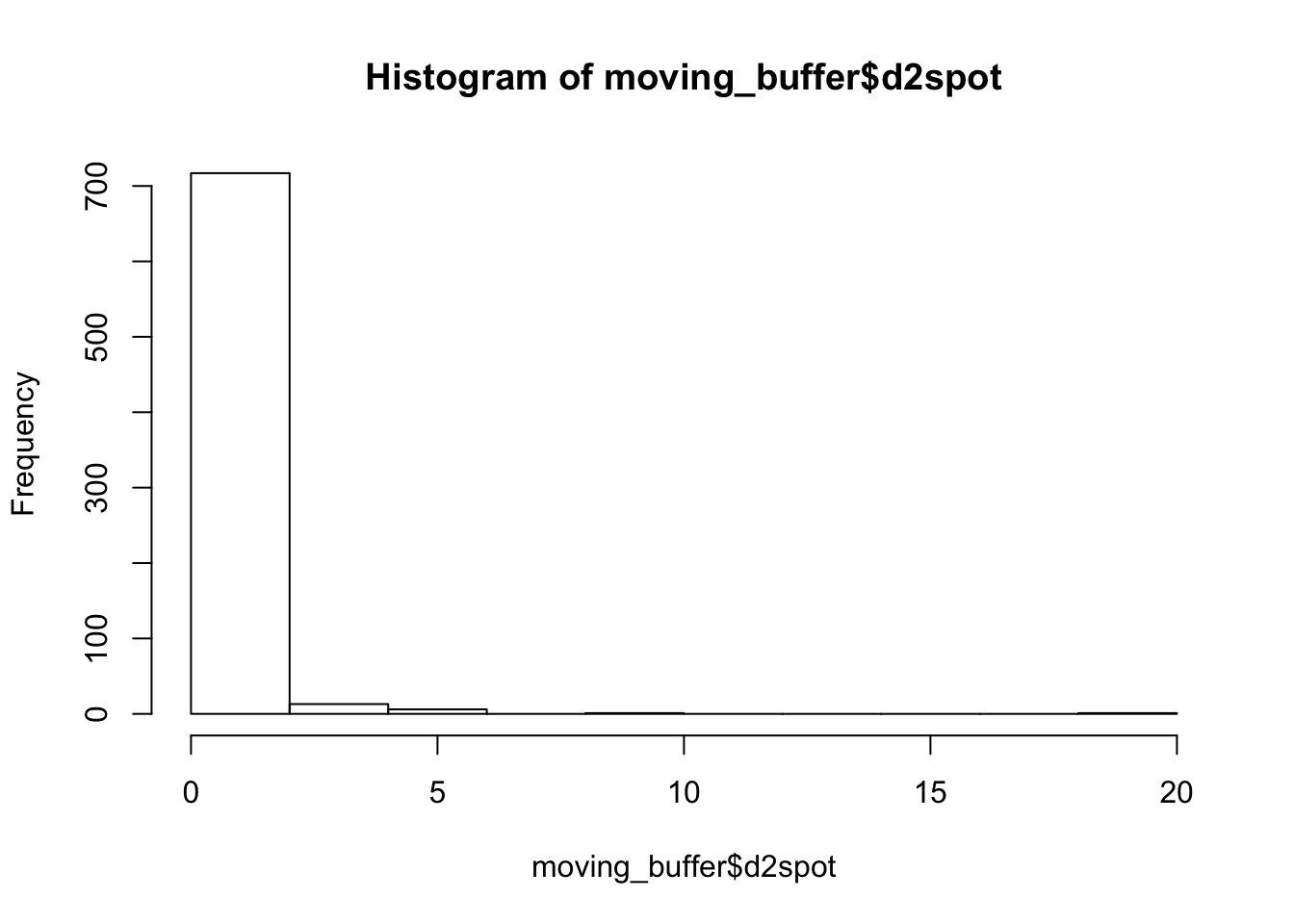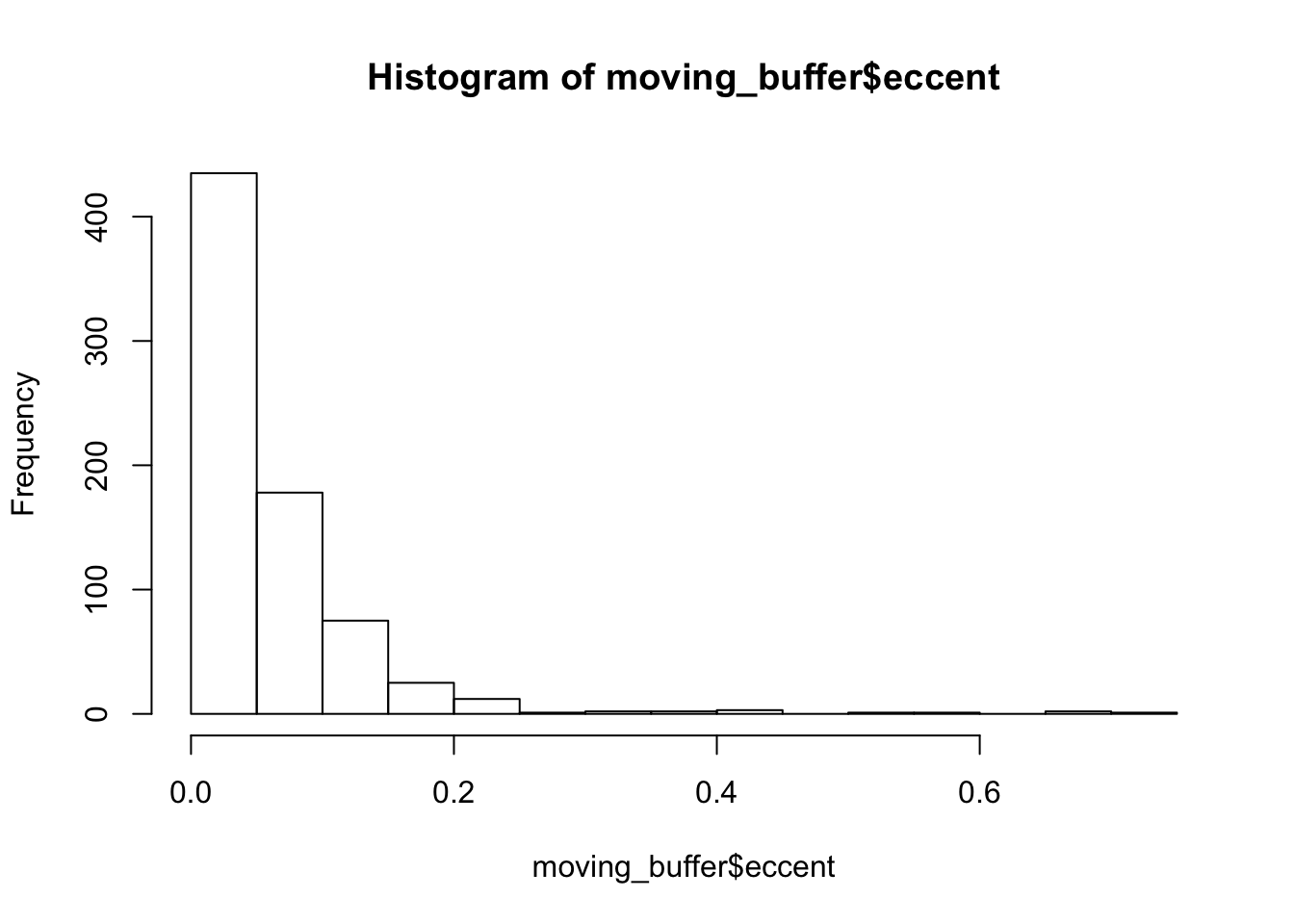# library(gsubfn)

``````rm(list=ls())
debug = 0;

# Take all the single cell traps

``Data.trap.1Cell = DataTrap[ DataTrap\$total_cells == 1, ]; ``

# calculathe the moving null distribution using single cell subset

``````moving_buffer = data.frame(matrix(data=NA, nrow = length(Data.trap.1Cell[,1]), ncol = 5));
names(moving_buffer) = c("d", "area", "d2spot", "eccent");

my_dist = function(x1, y1, x2, y2) {sqrt( (x1 - x2)^2 + (y1-y2)^2 ) };

#for( i in 2:length(Data.trap.1Cell[,1]) ){
for( i in 2:999 ){
previous = Data.trap.1Cell[ i-1, ];
current = Data.trap.1Cell [i,    ];

#check image number
if ( previous\$Image_num == current\$Image_num - 1 ) {
# prevous and current segments are right next to each other in the time series
if (debug > 0) { print(paste("calculate previous=", i-1, "current=",i )); }
moving_buffer\$d[i] = sqrt( (current\$cell_X - previous\$cell_X)^2 + (current\$cell_Y - previous\$cell_Y)^2 );
moving_buffer\$area[i]= abs(current\$cell_area - previous\$cell_area );
moving_buffer\$d2spot[i] = abs(current\$cellsSpot_Dist - previous\$cellsSpot_Dist );
moving_buffer\$eccent[i] = abs(current\$eccentricity - previous\$eccentricity );
} else { if (debug > 1 ) { print(paste("skip previous=", i-1, "current=",i )); }
}
}

summary(moving_buffer)``````
``````##        d               area            d2spot           eccent
##  Min.   : 0.000   Min.   : 0.000   Min.   : 0.000   Min.   :0.000
##  1st Qu.: 0.403   1st Qu.: 1.000   1st Qu.: 0.200   1st Qu.:0.017
##  Median : 0.608   Median : 2.000   Median : 0.400   Median :0.040
##  Mean   : 0.722   Mean   : 2.835   Mean   : 0.597   Mean   :0.060
##  3rd Qu.: 0.854   3rd Qu.: 3.000   3rd Qu.: 0.730   3rd Qu.:0.078
##  Max.   :14.403   Max.   :62.000   Max.   :19.500   Max.   :0.717
##  NA's   :8917     NA's   :8917     NA's   :8917     NA's   :8917
##     NA
##  Mode:logical
##  NA's:9655
##
##
##
##
## ``````
``hist( moving_buffer\$d );````hist( moving_buffer\$area )````hist( moving_buffer\$d2spot )````hist( moving_buffer\$eccent )``## p-value calculations

``````p_dist = function(x, input_buffer) {
input_buffer = input_buffer[ !is.na(input_buffer)];
lower = input_buffer[ input_buffer < x]; lower = lower[!is.na(lower)];
upper = input_buffer[input_buffer > x]; upper = upper[!is.na(upper)];

LowerP =  (length(lower) / length(input_buffer))
UpperP =  (length(upper) / length(input_buffer))

return(list(LowerP,UpperP, mean(input_buffer, na.rm=T), sd(input_buffer, na.rm=T)))
}

p_dist( 10, moving_buffer\$d)   #  lower and upper bound of  distance  probability for  one cell ``````
``````## []
##  0.998645
##
## []
##  0.001355014
##
## []
##  0.722106
##
## []
##  0.7843616``````

## Probability Density Function & cumulative Distribution Funcion for area

`` p_dist( 5, moving_buffer\$area)``
``````## []
##  0.8292683
##
## []
##  0.1056911
##
## []
##  2.834688
##
## []
##  4.117974``````

## Null distribution of cell distance from spot passed trap

``p_dist(10, moving_buffer\$d2spot)``
``````## []
##  0.998645
##
## []
##  0.001355014
##
## []
##  0.597019
##
## []
##  0.9887488``````
``p_dist(5, moving_buffer\$eccent)``
``````## []
##  1
##
## []
##  0
##
## []
##  0.06044309
##
## []
##  0.07491423``````
``write.csv(moving_buffer, "data/moving_buffer.csv", quote = F, row.names = F)``
Fit with gamma distribution
``````library(MASS)

x = moving_buffer\$d;
x[x==0] = 0.05; #zero should be adjusted to a small value.
x = x[!is.na(x)];
fitdistr(x, "log-normal");``````
``````##      meanlog        sdlog
##   -0.57420032    0.69299129
##  ( 0.02550936) ( 0.01803784)``````
``````x = moving_buffer\$area;
x[x==0] = 0.05;
x = x[!is.na(x)];
fitdistr(x, "log-normal");``````
``````##     meanlog       sdlog
##   0.27596012   1.54133093
##  (0.05673718) (0.04011924)``````
``````x = moving_buffer\$d2spot;
x[x==0] = 0.05;
x = x[!is.na(x)];
fitdistr(x, "log-normal");``````
``````##      meanlog        sdlog
##   -1.05515338    1.09676306
##  ( 0.04037241) ( 0.02854760)``````
``````x = moving_buffer\$eccent;
x[x==0] = 0.05;
x = x[!is.na(x)];
fitdistr(x, "log-normal");``````
``````##      meanlog        sdlog
##   -3.35301141    1.17329016
##  ( 0.04318941) ( 0.03053952)``````